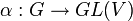# Continuous linear representation of topological group over topological field

Suppose$G$ is a topological group,$K$ is a topological field, and$V$ is a topological vector space over$K$. A continuous linear representation of$G$ over$V$ (and hence over$K$) is a linear representation$\alpha: G \to GL(V)$, such that, when viewed as a map$G \times V \to V$, it is jointly continuous. In other words, it is continuous from$G \times V$ (endowed with the product topology) to$V$.